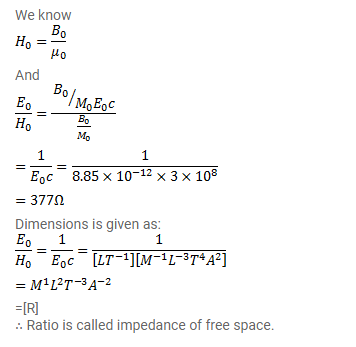# The value of

Question:

Using $B=\mu_{0} H_{\text {find the ratio }} E_{0} / H_{0}$ for a plane electromagnetic wave propagating through vacuum. Show that it has the dimension of electric resistance. This ratio is a universal constant called the impedance of free space.

Solution: